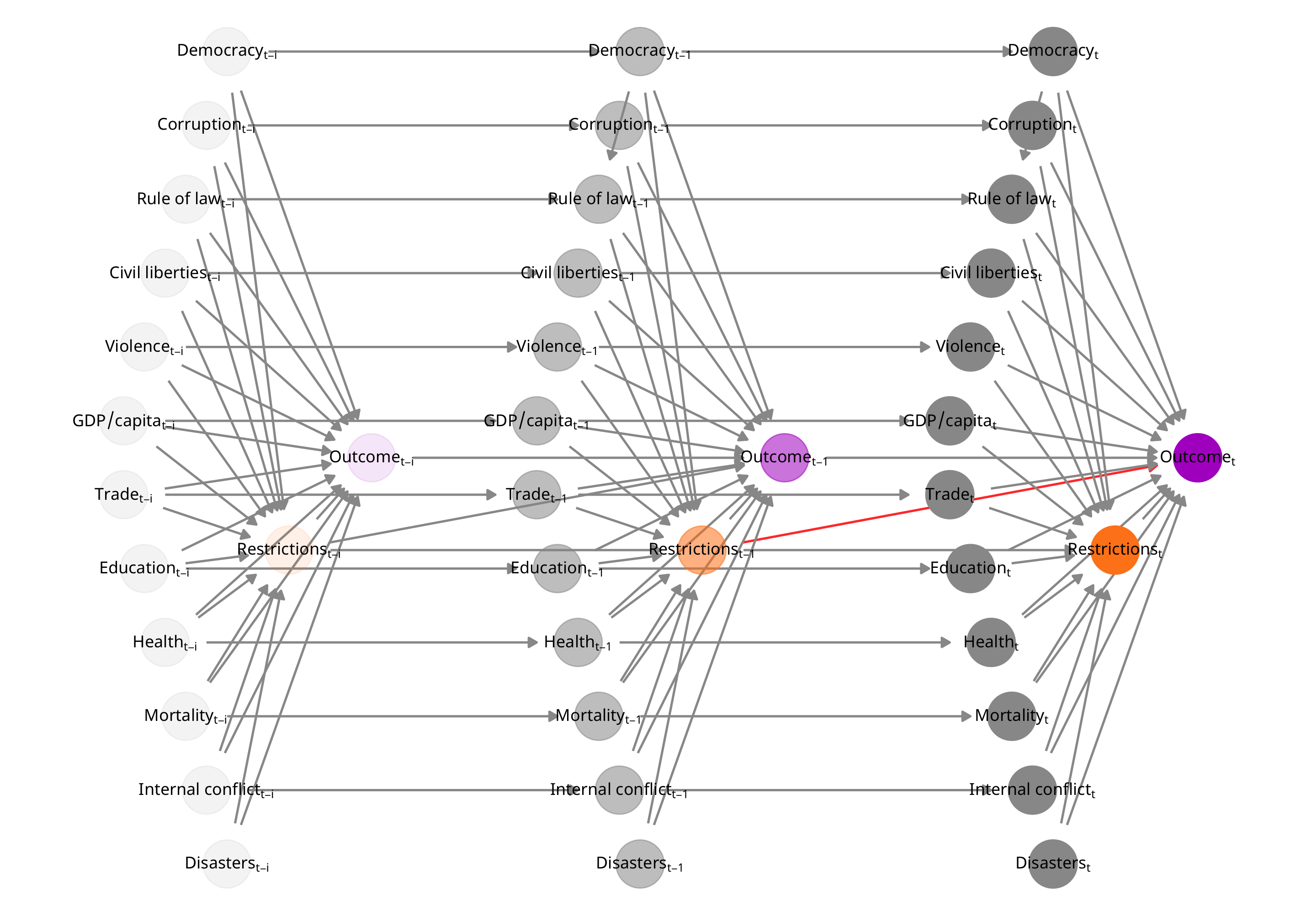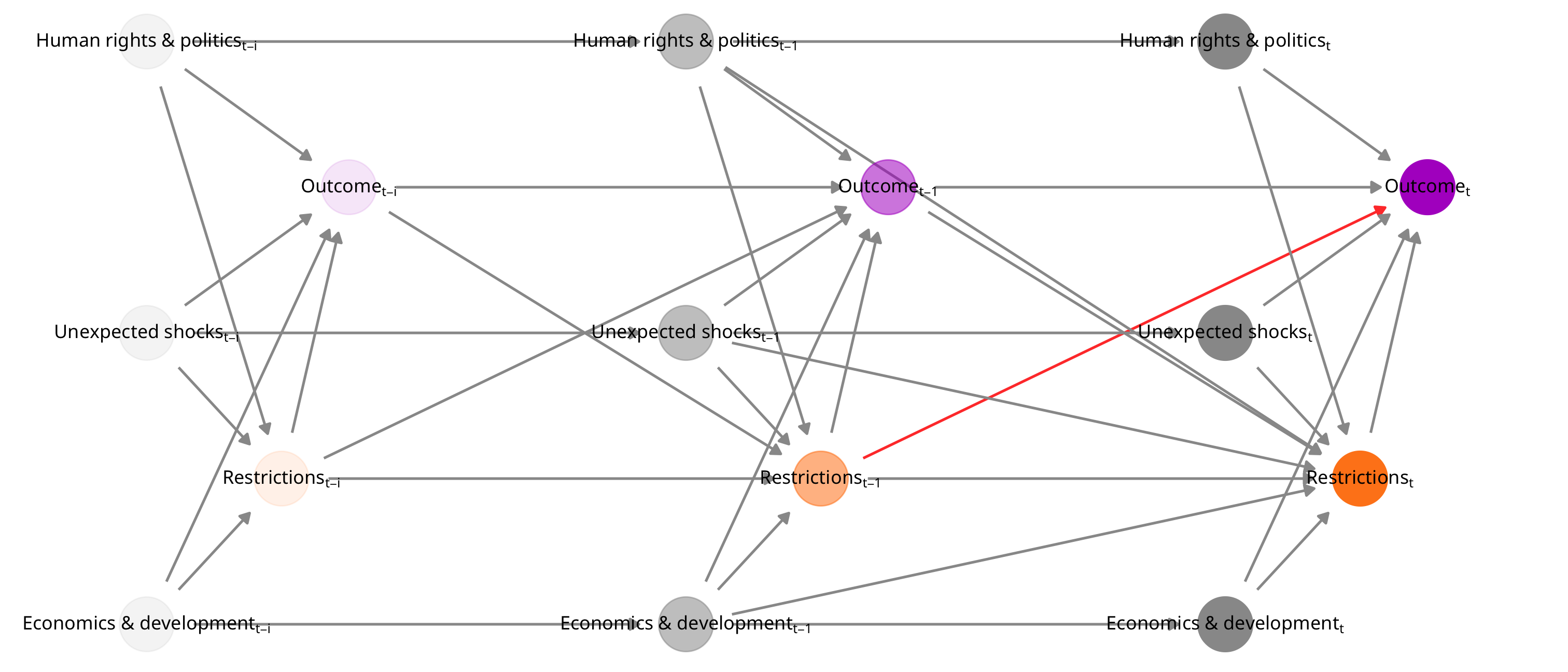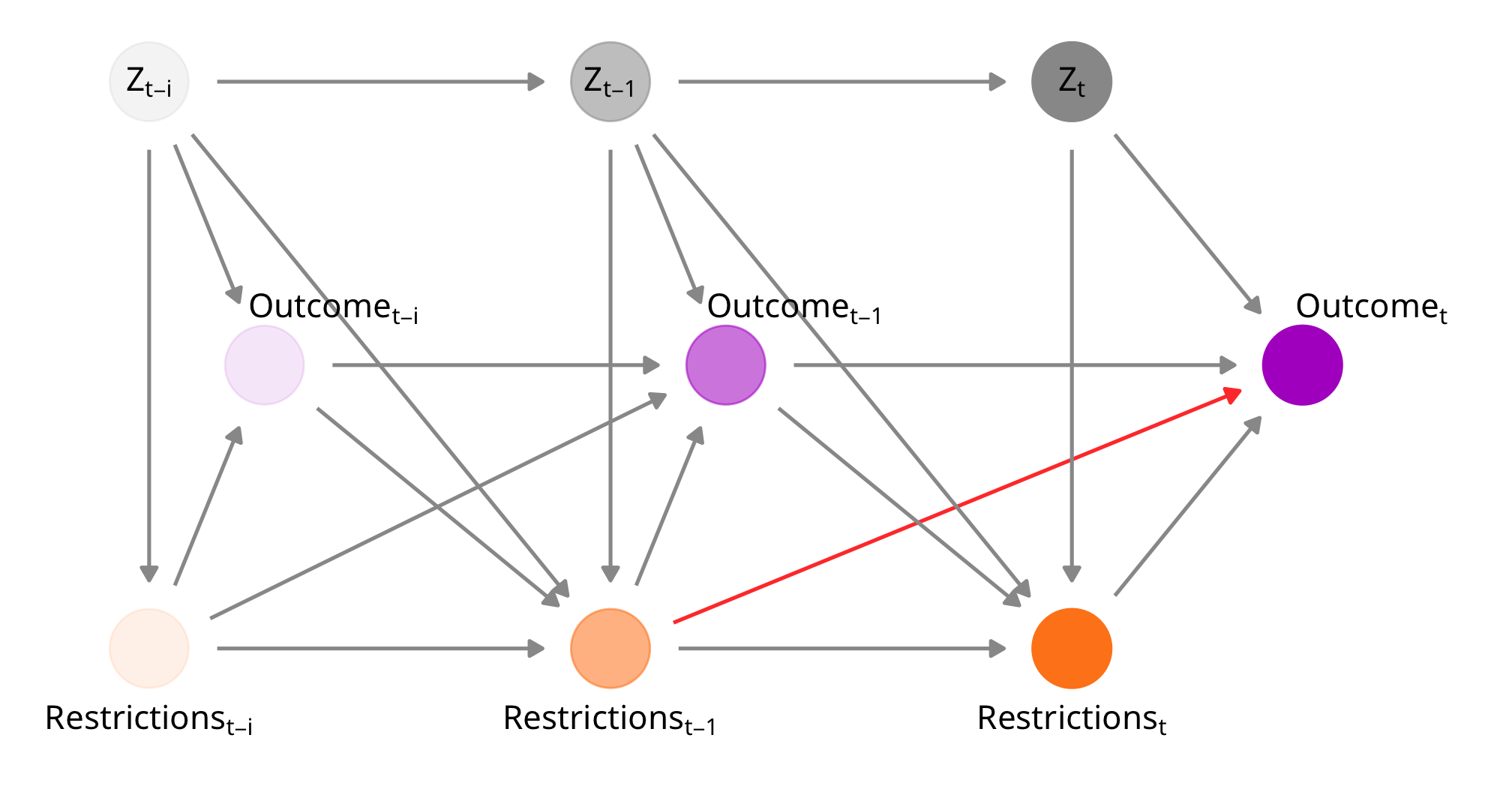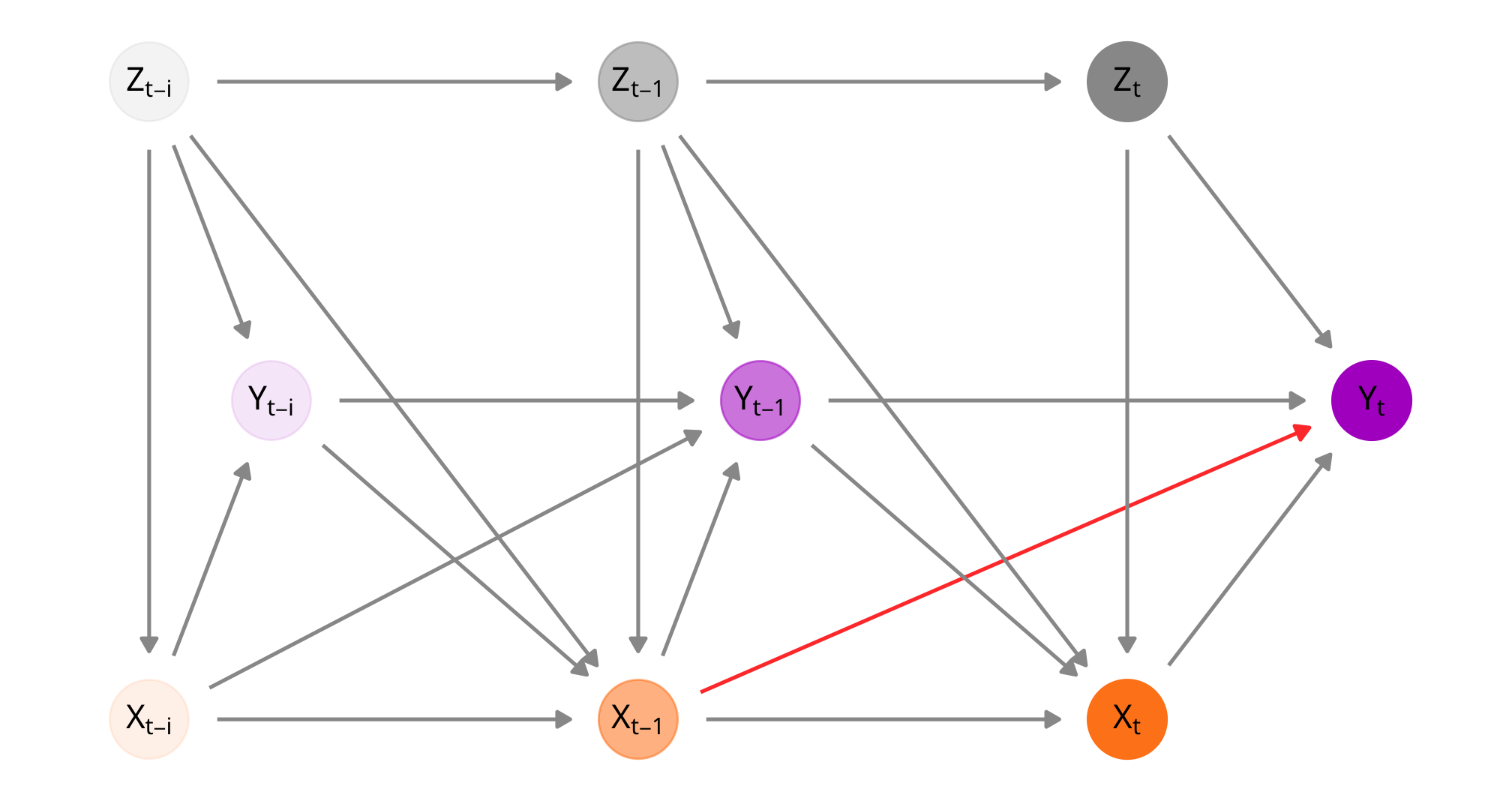library(tidyverse)
library(ggdag)
library(dagitty)
library(here)

source(here("lib", "graphics.R"))
source(here("lib", "misc_funs.R"))

update_geom_defaults(ggdag:::GeomDagText, list(family = "Noto Sans", face = "plain"))

my_seed <- 1234
set.seed(my_seed)

# Full messy model

huge_messy_dag <- dagitty('
dag {
"Corruption[t-i]" [pos="2.000,2.000"]
"Corruption[t]" [pos="12.000,2.000"]
"Democracy[t-i]" [pos="2.250,1.000"]
"Democracy[t]" [pos="12.250,1.000"]
"Disasters[t-i]" [pos="2.250,12.000"]
"Disasters[t]" [pos="12.250,12.000"]
"Education[t-i]" [pos="1.250,8.000"]
"Education[t]" [pos="11.250,8.000"]
"GDP/capita[t-i]" [pos="1.000,6.000"]
"GDP/capita[t]" [pos="11.000,6.000"]
"Health[t-i]" [pos="1.500,9.000"]
"Health[t]" [pos="11.500,9.000"]
"Mortality[t-i]" [pos="1.750,10.000"]
"Mortality[t]" [pos="11.750,10.000"]
"Outcome[t-1]" [pos="9.000,6.500"]
"Outcome[t-i]" [pos="4.000,6.500"]
"Outcome[t]" [outcome,pos="14.000,6.500"]
"Restrictions[t-1]" [exposure,pos="8.000,7.750"]
"Restrictions[t]" [pos="13.000,7.750"]
"Violence[t-i]" [pos="1.250,5.000"]
"Violence[t]" [pos="11.250,5.000"]
"Civil liberties[t-1]" [adjusted,pos="6.500,4.000"]
"Civil liberties[t-i]" [pos="1.500,4.000"]
"Civil liberties[t]" [pos="11.500,4.000"]
"Internal conflict[t-1]" [adjusted,pos="7.000,11.000"]
"Internal conflict[t-i]" [pos="2.000,11.000"]
"Internal conflict[t]" [pos="12.000,11.000"]
"Rule of law[t-1]" [adjusted,pos="6.750,3.000"]
"Rule of law[t-i]" [pos="1.750,3.000"]
"Rule of law[t]" [pos="11.750,3.000"]
"Corruption[t-1]" -> "Corruption[t]"
"Corruption[t-1]" -> "Outcome[t-1]"
"Corruption[t-1]" -> "Restrictions[t-1]"
"Corruption[t-i]" -> "Corruption[t-1]"
"Corruption[t-i]" -> "Outcome[t-i]"
"Corruption[t-i]" -> "Restrictions[t-i]"
"Corruption[t]" -> "Outcome[t]"
"Corruption[t]" -> "Restrictions[t]"
"Democracy[t-1]" -> "Corruption[t-1]"
"Democracy[t-1]" -> "Democracy[t]"
"Democracy[t-1]" -> "Outcome[t-1]"
"Democracy[t-1]" -> "Restrictions[t-1]"
"Democracy[t-1]" -> "Rule of law[t-1]"
"Democracy[t-i]" -> "Democracy[t-1]"
"Democracy[t-i]" -> "Outcome[t-i]"
"Democracy[t-i]" -> "Restrictions[t-i]"
"Democracy[t]" -> "Corruption[t]"
"Democracy[t]" -> "Outcome[t]"
"Democracy[t]" -> "Restrictions[t]"
"Democracy[t]" -> "Rule of law[t]"
"Disasters[t-1]" -> "Outcome[t-1]"
"Disasters[t-1]" -> "Restrictions[t-1]"
"Disasters[t-i]" -> "Outcome[t-i]"
"Disasters[t-i]" -> "Restrictions[t-i]"
"Disasters[t]" -> "Outcome[t]"
"Disasters[t]" -> "Restrictions[t]"
"Education[t-1]" -> "Education[t]"
"Education[t-1]" -> "Outcome[t-1]"
"Education[t-1]" -> "Restrictions[t-1]"
"Education[t-i]" -> "Education[t-1]"
"Education[t-i]" -> "Outcome[t-i]"
"Education[t-i]" -> "Restrictions[t-i]"
"Education[t]" -> "Outcome[t]"
"Education[t]" -> "Restrictions[t]"
"GDP/capita[t-1]" -> "GDP/capita[t]"
"GDP/capita[t-1]" -> "Outcome[t-1]"
"GDP/capita[t-1]" -> "Restrictions[t-1]"
"GDP/capita[t-i]" -> "GDP/capita[t-1]"
"GDP/capita[t-i]" -> "Outcome[t-i]"
"GDP/capita[t-i]" -> "Restrictions[t-i]"
"GDP/capita[t]" -> "Outcome[t]"
"GDP/capita[t]" -> "Restrictions[t]"
"Health[t-1]" -> "Health[t]"
"Health[t-1]" -> "Outcome[t-1]"
"Health[t-1]" -> "Restrictions[t-1]"
"Health[t-i]" -> "Health[t-1]"
"Health[t-i]" -> "Outcome[t-i]"
"Health[t-i]" -> "Restrictions[t-i]"
"Health[t]" -> "Outcome[t]"
"Health[t]" -> "Restrictions[t]"
"Mortality[t-1]" -> "Mortality[t]"
"Mortality[t-1]" -> "Outcome[t-1]"
"Mortality[t-1]" -> "Restrictions[t-1]"
"Mortality[t-i]" -> "Mortality[t-1]"
"Mortality[t-i]" -> "Outcome[t-i]"
"Mortality[t-i]" -> "Restrictions[t-i]"
"Mortality[t]" -> "Outcome[t]"
"Mortality[t]" -> "Restrictions[t]"
"Outcome[t-1]" -> "Outcome[t]"
"Outcome[t-i]" -> "Outcome[t-1]"
"Restrictions[t-1]" -> "Outcome[t-1]"
"Restrictions[t-1]" -> "Outcome[t]"
"Restrictions[t-1]" -> "Restrictions[t]"
"Restrictions[t-i]" -> "Outcome[t-1]"
"Restrictions[t-i]" -> "Outcome[t-i]"
"Restrictions[t-i]" -> "Restrictions[t-1]"
"Restrictions[t]" -> "Outcome[t]"
"Violence[t-1]" -> "Outcome[t-1]"
"Violence[t-1]" -> "Restrictions[t-1]"
"Violence[t-1]" -> "Violence[t]"
"Violence[t-i]" -> "Outcome[t-i]"
"Violence[t-i]" -> "Restrictions[t-i]"
"Violence[t-i]" -> "Violence[t-1]"
"Violence[t]" -> "Outcome[t]"
"Violence[t]" -> "Restrictions[t]"
"Civil liberties[t-1]" -> "Outcome[t-1]"
"Civil liberties[t-1]" -> "Restrictions[t-1]"
"Civil liberties[t-1]" -> "Civil liberties[t]"
"Civil liberties[t-i]" -> "Outcome[t-i]"
"Civil liberties[t-i]" -> "Restrictions[t-i]"
"Civil liberties[t-i]" -> "Civil liberties[t-1]"
"Civil liberties[t]" -> "Outcome[t]"
"Civil liberties[t]" -> "Restrictions[t]"
"Internal conflict[t-1]" -> "Outcome[t-1]"
"Internal conflict[t-1]" -> "Restrictions[t-1]"
"Internal conflict[t-1]" -> "Internal conflict[t]"
"Internal conflict[t-i]" -> "Outcome[t-i]"
"Internal conflict[t-i]" -> "Restrictions[t-i]"
"Internal conflict[t-i]" -> "Internal conflict[t-1]"
"Internal conflict[t]" -> "Outcome[t]"
"Internal conflict[t]" -> "Restrictions[t]"
"Rule of law[t-1]" -> "Outcome[t-1]"
"Rule of law[t-1]" -> "Restrictions[t-1]"
"Rule of law[t-1]" -> "Rule of law[t]"
"Rule of law[t-i]" -> "Outcome[t-i]"
"Rule of law[t-i]" -> "Restrictions[t-i]"
"Rule of law[t-i]" -> "Rule of law[t-1]"
"Rule of law[t]" -> "Outcome[t]"
"Rule of law[t]" -> "Restrictions[t]"
}
')

huge_messy_dag_plot <- huge_messy_dag %>%
tidy_dagitty() %>%
mutate(var_type = case_when(
str_detect(name, "Outcome") ~ "Outcome",
str_detect(name, "Restrictions") ~ "Restrictions",
TRUE ~ "Z"
)) %>%
mutate(time_period = case_when(
str_detect(name, "t-1") ~ 2,
str_detect(name, "t-i") ~ 1,
TRUE ~ 3
)) %>%
mutate(arrow_color = case_when(
name == "Restrictions[t-1]" & to == "Outcome[t]" ~ "#FF4136",
TRUE ~ "grey60"
))

ggplot(huge_messy_dag_plot, aes(x = x, y = y, xend = xend, yend = yend)) +
geom_dag_edges(aes(edge_colour = arrow_color)) +
geom_dag_point(aes(color = var_type, alpha = time_period), size = 12) +
geom_dag_text(data = filter(huge_messy_dag_plot, var_type == "Outcome"),
color = "black", size = pts(9), parse = TRUE) +
geom_dag_text(data = filter(huge_messy_dag_plot, var_type == "Restrictions"),
color = "black", size = pts(9), parse = TRUE) +
geom_dag_text(data = filter(huge_messy_dag_plot, var_type == "Z"),
color = "black", size = pts(9), parse = TRUE) +
scale_color_manual(values = c("#B10DC9", "#FF851B", "grey60")) +
scale_y_reverse() +
guides(alpha = FALSE, color = FALSE) +
theme_dag()# Simpler model with families of confounders

simplerish_dag <- dagitty('
dag {
"Human rights & politics[t-1]" [adjusted,pos="5,1.5"]
"Human rights & politics[t-i]" [pos="1,1.5"]
"Human rights & politics[t]" [pos="9,1.5"]
"Economics & development[t-1]" [adjusted,pos="5,2.5"]
"Economics & development[t-i]" [pos="1,2.5"]
"Economics & development[t]" [pos="9,2.5"]
"Outcome[t-1]" [pos="6.5,1.75"]
"Outcome[t]" [outcome,pos="10.5,1.75"]
"Restrictions[t-1]" [exposure,pos="6,2.25"]
"Restrictions[t]" [pos="10,2.25"]
"Unexpected shocks[t-1]" [adjusted,pos="5,2"]
"Unexpected shocks[t-i]" [pos="1,2"]
"Unexpected shocks[t]" [pos="9,2"]
"Human rights & politics[t-1]" -> "Human rights & politics[t]"
"Human rights & politics[t-1]" -> "Outcome[t-1]"
"Human rights & politics[t-1]" -> "Restrictions[t-1]"
"Human rights & politics[t-1]" -> "Restrictions[t]"
"Human rights & politics[t-i]" -> "Human rights & politics[t-1]"
"Human rights & politics[t-i]" -> "Outcome[t-i]"
"Human rights & politics[t-i]" -> "Restrictions[t-i]"
"Human rights & politics[t]" -> "Outcome[t]"
"Human rights & politics[t]" -> "Restrictions[t]"
"Economics & development[t-1]" -> "Economics & development[t]"
"Economics & development[t-1]" -> "Outcome[t-1]"
"Economics & development[t-1]" -> "Restrictions[t-1]"
"Economics & development[t-1]" -> "Restrictions[t]"
"Economics & development[t-i]" -> "Economics & development[t-1]"
"Economics & development[t-i]" -> "Outcome[t-i]"
"Economics & development[t-i]" -> "Restrictions[t-i]"
"Economics & development[t]" -> "Outcome[t]"
"Economics & development[t]" -> "Restrictions[t]"
"Outcome[t-1]" -> "Outcome[t]"
"Outcome[t-1]" -> "Restrictions[t]"
"Outcome[t-i]" -> "Outcome[t-1]"
"Outcome[t-i]" -> "Restrictions[t-1]"
"Restrictions[t]" -> "Outcome[t]"
"Restrictions[t-1]" -> "Outcome[t-1]"
"Restrictions[t-i]" -> "Outcome[t-i]"
"Restrictions[t-1]" -> "Outcome[t]"
"Restrictions[t-1]" -> "Restrictions[t]"
"Restrictions[t-i]" -> "Outcome[t-1]"
"Restrictions[t-i]" -> "Restrictions[t-1]"
"Unexpected shocks[t-1]" -> "Outcome[t-1]"
"Unexpected shocks[t-1]" -> "Restrictions[t-1]"
"Unexpected shocks[t-1]" -> "Restrictions[t]"
"Unexpected shocks[t-1]" -> "Unexpected shocks[t]"
"Unexpected shocks[t-i]" -> "Outcome[t-i]"
"Unexpected shocks[t-i]" -> "Restrictions[t-i]"
"Unexpected shocks[t-i]" -> "Unexpected shocks[t-1]"
"Unexpected shocks[t]" -> "Outcome[t]"
"Unexpected shocks[t]" -> "Restrictions[t]"
}
')

simplerish_dag_plot <- simplerish_dag %>%
tidy_dagitty() %>%
mutate(var_type = case_when(
str_detect(name, "Outcome") ~ "Outcome",
str_detect(name, "Restrictions") ~ "Restrictions",
TRUE ~ "Z"
)) %>%
mutate(time_period = case_when(
str_detect(name, "t-1") ~ 2,
str_detect(name, "t-i") ~ 1,
TRUE ~ 3
)) %>%
mutate(arrow_color = case_when(
name == "Restrictions[t-1]" & to == "Outcome[t]" ~ "#FF4136",
TRUE ~ "grey60"
))

ggplot(simplerish_dag_plot, aes(x = x, y = y, xend = xend, yend = yend)) +
geom_dag_edges(aes(edge_colour = arrow_color)) +
geom_dag_point(aes(color = var_type, alpha = time_period), size = 12) +
geom_dag_text(data = filter(simplerish_dag_plot, var_type == "Outcome"),
color = "black", size = pts(9), parse = TRUE) +
geom_dag_text(data = filter(simplerish_dag_plot, var_type == "Restrictions"),
color = "black", size = pts(9), parse = TRUE) +
geom_dag_text(data = filter(simplerish_dag_plot, var_type == "Z"),
color = "black", size = pts(9), parse = TRUE) +
scale_color_manual(values = c("#B10DC9", "#FF851B", "grey60")) +
scale_y_reverse() +
guides(alpha = FALSE, color = FALSE) +
theme_dag()# Simplest model

simple_dag <- dagitty('
dag {
"Outcome[t-i]" [pos="1.5,2"]
"Outcome[t-1]" [pos="3.5,2"]
"Outcome[t]" [outcome,pos="6,2"]
"Restrictions[t-i]" [pos="1,1"]
"Restrictions[t-1]" [exposure,pos="3,1"]
"Restrictions[t]" [pos="5,1"]
"Z[t-i]" [pos="1,3"]
"Z[t-1]" [pos="3,3"]
"Z[t]" [pos="5,3"]
"Outcome[t-1]" -> "Outcome[t]"
"Outcome[t-1]" -> "Restrictions[t]"
"Outcome[t-i]" -> "Outcome[t-1]"
"Outcome[t-i]" -> "Restrictions[t-1]"
"Restrictions[t]" -> "Outcome[t]"
"Restrictions[t-1]" -> "Outcome[t-1]"
"Restrictions[t-i]" -> "Outcome[t-i]"
"Restrictions[t-1]" -> "Outcome[t]"
"Restrictions[t-1]" -> "Restrictions[t]"
"Restrictions[t-i]" -> "Outcome[t-1]"
"Restrictions[t-i]" -> "Restrictions[t-1]"
"Z[t-1]" -> "Outcome[t-1]"
"Z[t-1]" -> "Restrictions[t-1]"
"Z[t-1]" -> "Restrictions[t]"
"Z[t-1]" -> "Z[t]"
"Z[t-i]" -> "Outcome[t-i]"
"Z[t-i]" -> "Restrictions[t-1]"
"Z[t-i]" -> "Restrictions[t-i]"
"Z[t-i]" -> "Z[t-1]"
"Z[t]" -> "Outcome[t]"
"Z[t]" -> "Restrictions[t]"
}
')

simple_dag_plot <- simple_dag %>%
tidy_dagitty() %>%
mutate(var_type = case_when(
str_detect(name, "Outcome") ~ "Outcome",
str_detect(name, "Restrictions") ~ "Restrictions",
str_detect(name, "Z") ~ "Z"
)) %>%
mutate(time_period = case_when(
str_detect(name, "t-1") ~ 2,
str_detect(name, "t-i") ~ 1,
TRUE ~ 3
)) %>%
mutate(arrow_color = case_when(
name == "Restrictions[t-1]" & to == "Outcome[t]" ~ "#FF4136",
TRUE ~ "grey60"
)) %>%
mutate(letter_only = case_when(
str_detect(name, "Outcome") ~ str_replace(name, "Outcome", "Y"),
str_detect(name, "Restrictions") ~ str_replace(name, "Restrictions", "X"),
TRUE ~ name
))

simple_dag_out <- ggplot(simple_dag_plot, aes(x = x, y = y, xend = xend, yend = yend)) +
geom_dag_edges(aes(edge_colour = arrow_color)) +
geom_dag_point(aes(color = var_type, alpha = time_period), size = 12) +
geom_dag_text(data = filter(simple_dag_plot, var_type == "Outcome"),
color = "black", size = pts(11), parse = TRUE,
nudge_y = 0.2, nudge_x = 0.3) +
geom_dag_text(data = filter(simple_dag_plot, var_type == "Restrictions"),
color = "black", size = pts(11), parse = TRUE,
nudge_y = -0.25) +
geom_dag_text(data = filter(simple_dag_plot, var_type == "Z"),
color = "black", size = pts(11), parse = TRUE,
nudge_y = 0) +
scale_color_manual(values = c("#B10DC9", "#FF851B", "grey60")) +
guides(alpha = FALSE, color = FALSE) +
theme_dag()
simple_dag_outsimple_dag_letters_out <- ggplot(simple_dag_plot, aes(x = x, y = y, xend = xend, yend = yend)) +
geom_dag_edges(aes(edge_colour = arrow_color)) +
geom_dag_point(aes(color = var_type, alpha = time_period), size = 12) +
geom_dag_text(aes(label = letter_only),
color = "black", size = pts(11), parse = TRUE) +
scale_color_manual(values = c("#B10DC9", "#FF851B", "grey60")) +
guides(alpha = FALSE, color = FALSE) +
theme_dag()
simple_dag_letters_outggsave(here("analysis", "output", "dag_simple_letters.pdf"), simple_dag_letters_out,
width = 7, height = 3.75, device = cairo_pdf)
ggsave(here("analysis", "output", "dag_simple_letters.png"), simple_dag_letters_out,
width = 7, height = 3.75, dpi = 300, type = "cairo")

These DAGs are complex—especially the one with every possible node. However, they have important analytical value. We cannot randomly assign countries to impose anti-NGO laws or restrict the environment for civil society—doing that is completely infeasable (and unethical!). This means that we cannot use experimental data to measure the causal effect of anti-NGO restrictions on foreign aid, or $$E[\text{Aid} \mid do(\text{Restrictions})]$$. We also don’t have any quasi-experimental situations that would allow for context-based identification of the effect of restrictions on aid.

Simply measuring $$E[\text{Aid} \mid \text{Restrictions}]$$ is trivial—just run lm(aid ~ restrictions) and look at the coefficient. However, this estimate cannot be interpreted in any sort of causal way, since correlation is not causation. Using the logic of do-calculus, we can actually use the relationships between the nodes in the DAG to transform $$E[\text{Aid} \mid do(\text{Restrictions})]$$ into a do-free expression by making statistical adjustments and closing backdoor pathways that open up spurious relationships between restrictions and aid. As long as we can find a minimally sufficient adjustment set—or a set of nodes or variables that need to be adjusted in order to ensure that restrictions is d-separated from aid—we can isolate and identify the causal link between restrictions and aid.

Rather than go through the complex math behind the three rules of do-calculus, we can use R (or dagitty.net) to identify minimal sufficient adjustment sets based on the three DAGs above.

• Huge complicated DAG:

huge_messy_dag %>%
adjustmentSets()
## { Corruption[t-1], Democracy[t-1], Disasters[t-1], Education[t-1],
##   GDP/capita[t-1], Health[t-1], Mortality[t-1], Restrictions[t-i], Trade[t-1],
##   Violence[t-1], Civil liberties[t-1], Internal conflict[t-1], Rule of
##   law[t-1] }
• Simpler-ish DAG:

simplerish_dag %>%
adjustmentSets()
## { Outcome[t-i], Restrictions[t-i], Economics & development[t-1], Human rights
##   & politics[t-1], Unexpected shocks[t-1] }
• Simple DAG:

simple_dag %>%
adjustmentSets()
## { Outcome[t-i], Restrictions[t-i], Z[t-1] }

In other words, if we want to isolate the causal effect of anti-NGO restrictions in time $$t$$ on aid in time $$t+1$$ (i.e. the effect of lagged restrictions), we need to adjust for lagged aid ($$t-1$$), lagged restrictions ($$t-1$$), and time-varying confounders ($$t$$).

Fortunately, marginal structural models allow us to make all of these adjustments using special inverse probability weights that take this lagging structure and treatment history into account.

$\text{Continuous stabilized IPW}_{it} = \prod^t_{t = 1} \frac{f_{X | \bar{X}, V}[(X_{it} | \bar{X}_{i, t-1}, V_i); \mu_1, \sigma^2_1]}{f_{X | \bar{X}, Y, Z, V}[(X_{it} | \bar{X}_{i, t-1}, Y_{i, t-1}, Z_{it}, V_i), \mu_2, \sigma^2_2]}$

• The numerator contains the probability of the observed treatment status ($$X_{it}$$) at each time given the previous history of treatment ($$\bar{X}_{i, t-1}$$) and time invariant confounders ($$V_i$$).
• The denominator contains the probability of the observed treatment status ($$X_{it}$$) at each time given the previous history of treatment ($$\bar{X}_{i, t-1}$$), previous outcome ($$Y_{i, t-1}$$), time varying confounders ($$Z_{it}$$), and time invariant confounders ($$V_i$$).
• These two probability distributions have a mean of $$\mu_{1, 2}$$ and variance of $$\sigma^2_{1, 2}$$.

Finally, these weights are used in an outcome model similar to this:

lm(outcome ~ lag_treatment, weights = ipw)

Doing this process closes the backdoor pathways between lagged restrictions and present aid and is theoretically sufficient for isolating the causal link between the two.

# Final variables to use

Based on these DAGs, here are the variables we use in our treatment and outcome models:

• Outcome
• H1: log aid in $$t$$ (total_oda_lead and total_oda_log_lead)
• H2: proportion of aid given for contentious causes in $$t$$ (prop_contentious)
• H3: proportion of aid given to domestic/international NGOS in $$t$$ (prop_ngo_dom and prop_ngo_foreign)
• Treatment
• Anti-civil society laws and general restrictive environment in $$t-1$$ (barriers_total and v2xcs_ccsi)
• Time-varying confounders (all in $$t-1$$)
• Human rights and politics
• Electoral democracy: v2x_polyarchy
• Corruption: v2x_corr
• Rule of law: v2x_rule
• Civil liberties index: v2x_civlib
• Physical violence index: v2x_clphy
• Private civil liberties index: v2x_clpriv
• Economics and development
• Wealth: gdpcap_log
• International trade: un_trade_pct_gdp
• Educational equality: v2peedueq
• Health equality: v2pehealth
• Infant mortality rate: e_peinfmor
• Unexpected shocks
• Internal conflict: internal_conflict_past_5
• Natural disasters: natural_dis_count
• Time-invarying confounders
• Country code included as multilevel random effects((1 | gwcode))